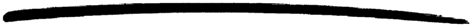# snmpa_local_db

snmpa_local_db

### MODULE SUMMARY

The SNMP built-in database

### DESCRIPTION

The module snmpa_local_db contains functions for implementing tables (and variables) using the SNMP built-in database. The database exists in two instances, one volatile and one persistent. The volatile database is implemented with ets. The persistent database is implemented with dets.

There is a scaling problem with this database.

• Insertions and deletions are inefficient for large tables.

This problem is best solved by using Mnesia instead.

The following functions describe the interface to snmpa_local_db. Each function has a Mnesia equivalent. The argument NameDb is a tuple {Name, Db} where Name is the symbolic name of the managed object (as defined in the MIB), and Db is either volatile or persistent. mnesia is not possible since all these functions are snmpa_local_db specific.

### Common Data Types

In the functions defined below, the following types are used:

• NameDb = {Name, Db}
• Name = atom(), Db = volatile | persistent
• RowIndex = [int()]
• Cols = [Col] | [{Col, Value}], Col = int(), Value = term()

where RowIndex denotes the last part of the OID, that specifies the index of the row in the table. Cols is a list of column numbers in case of a get operation, and a list of column numbers and values in case of a set operation.

### EXPORTS

Types:

Reason = term()

This function can be used to manually dump the database to file.

Performs an ets/dets matching on the table. See Stdlib documentation, module ets, for a description of Pattern and the return values.

Types:

TableName = atom()

Prints the contents of the database on screen. This is useful for debugging since the STANDARD-MIB and OTP-SNMPEA-MIB (and maybe your own MIBs) are stored in snmpa_local_db.

TableName is an atom for a table in the database. When no name is supplied, the whole database is shown.

Creates a table. If the table already exist, the old copy is destroyed.

Returns false if the NameDb argument is incorrectly specified, true otherwise.

Types:

Row = {Val1, Val2, ..., ValN}
Val1 = Val2 = ... = ValN = term()

Creates a row in a table. Row is a tuple with values for all columns, including the index columns.

Deletes a table.

Deletes the row in the table.

Checks if a table exists.

Types:

Row = {Val1, Val2, ..., ValN}
Val1 = Val2 = ... = ValN = term()

Row is a tuple with values for all columns, including the index columns.